#### Programmatically explore, debug, and manipulate ggplot internals### Installation

You can install the development version from GitHub with:

# install.packages("remotes")
remotes::install_github("yjunechoe/ggtrace")

library(ggtrace)

More on the 📦 package website: https://yjunechoe.github.io/ggtrace

### Description

ggtrace embodies an opinionated approach to learning about ggplot internals. The internals is a difficult topic, so I recommend watching the following presentations on ggtrace before getting started on any kind of code:

Read more about the philosophy behind ggtrace in the Getting Started vignette, and see examples in the Overview vignette.

ggtrace now also has a paper! Sub-layer modularity in the Grammar of Graphics

## Example usage

library(ggplot2)
packageVersion("ggplot2")
#>  '3.4.1'

### 1) Inspect sub-layer data

A bar plot of counts with geom_bar() with stat = "count" default:

bar_plot <- ggplot(mpg, aes(class, fill = class)) +
geom_bar() +
theme(legend.position = "none")

State of bar layer’s data after the statistical transformation step:

ggtrace::layer_after_stat(bar_plot)
#> ✔ Executed ggtrace_inspect_return(bar_plot, ggplot2:::Layer$compute_statistic) #> # A tibble: 7 × 8 #> count prop x width flipped_aes fill PANEL group #> <dbl> <dbl> <mppd_dsc> <dbl> <lgl> <chr> <fct> <int> #> 1 5 1 1 0.9 FALSE 2seater 1 1 #> 2 47 1 2 0.9 FALSE compact 1 2 #> 3 41 1 3 0.9 FALSE midsize 1 3 #> 4 11 1 4 0.9 FALSE minivan 1 4 #> 5 33 1 5 0.9 FALSE pickup 1 5 #> 6 35 1 6 0.9 FALSE subcompact 1 6 #> 7 62 1 7 0.9 FALSE suv 1 7 We can map aesthetics to variables from the after-stat data using after_stat(): bar_plot + geom_text( aes(label = after_stat(count)), stat = "count", position = position_nudge(y = 1), vjust = 0 )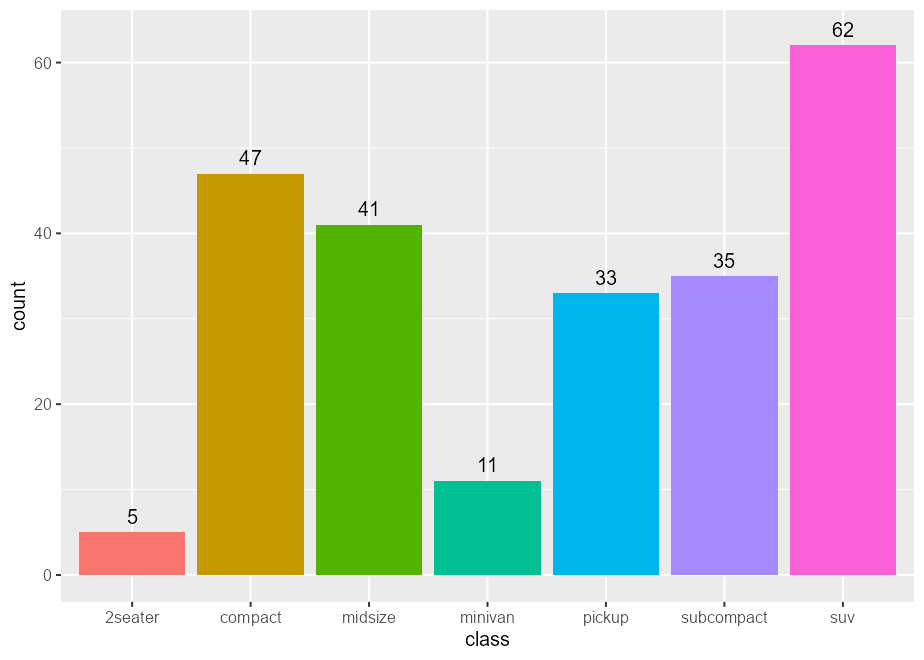Same idea with after_scale(): scatter_plot <- ggplot(mpg, aes(displ, hwy, fill = class)) + scale_fill_viridis_d(option = "magma") scatter_plot + geom_point(shape = 21, size = 4, stroke = 1)# fill column available for after_scale(fill) ggtrace::layer_after_scale(scatter_plot) #> ✔ Executed ggtrace_inspect_return(scatter_plot, ggplot2:::Layer$compute_geom_2)
#> # A tibble: 234 × 5
#>    fill          x     y PANEL group
#>    <chr>     <dbl> <dbl> <fct> <int>
#>  1 #2D1160FF   1.8    29 1         2
#>  2 #2D1160FF   1.8    29 1         2
#>  3 #2D1160FF   2      31 1         2
#>  4 #2D1160FF   2      30 1         2
#>  5 #2D1160FF   2.8    26 1         2
#>  6 #2D1160FF   2.8    26 1         2
#>  7 #2D1160FF   3.1    27 1         2
#>  8 #2D1160FF   1.8    26 1         2
#>  9 #2D1160FF   1.8    25 1         2
#> 10 #2D1160FF   2      28 1         2
#> # … with 224 more rows

scatter_plot +
geom_point(
aes(color = after_scale(prismatic::best_contrast(fill))),
shape = 21, size = 4, stroke = 1
)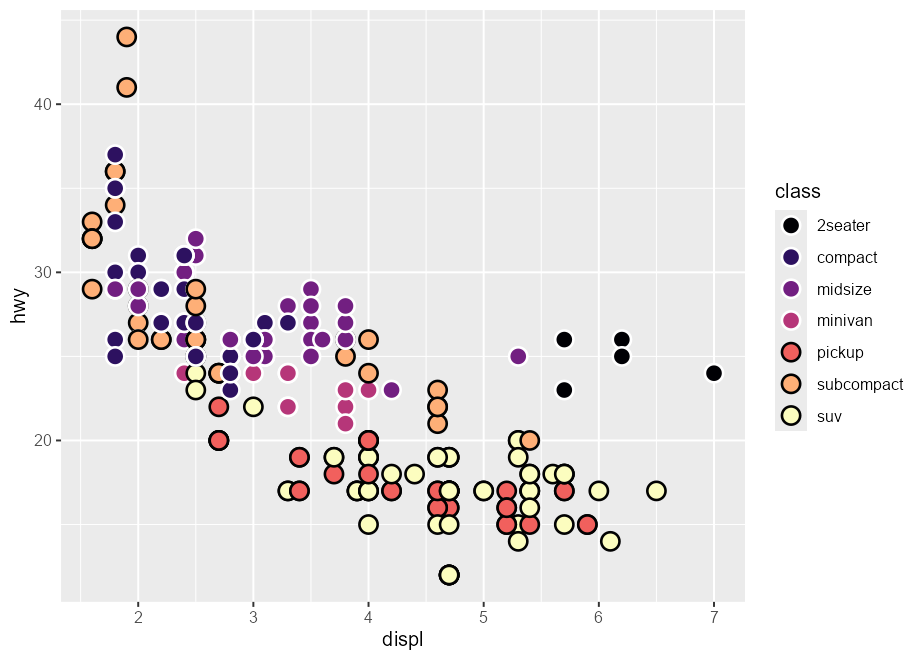### 2) Highjack ggproto (remove boxplot outliers)

You can hide outliers in geom_boxplot(), but they’ll still be in the layer’s underlying dataframe representation. This makes the plot look stretched:

boxplot_plot <- ggplot(mpg, aes(hwy, class)) +
geom_boxplot(outlier.shape = NA)
boxplot_plot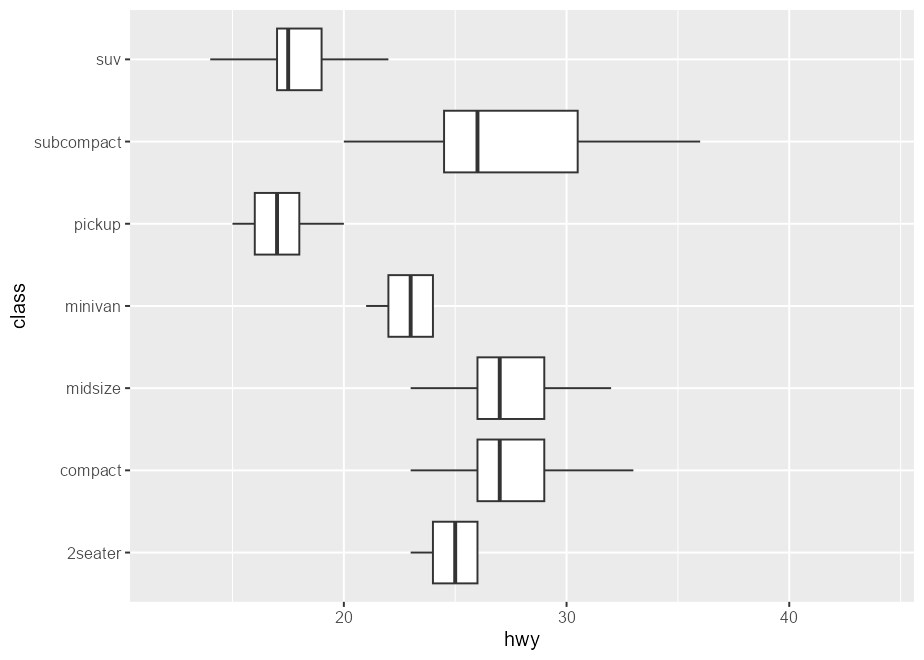This is because the scales are re-trained after the calculation of the boxplot statistics. In other words, the “final” min/max value of the x-scale are derived from the calculated outliers, even if they’re not drawn.

layer_data(boxplot_plot)[, c("xmin", "xmax", "outliers", "xmin_final", "xmax_final")]
#>   xmin xmax                       outliers xmin_final xmax_final
#> 1   23   26                                        23         26
#> 2   23   33                 35, 37, 35, 44         23         44
#> 3   23   32                                        23         32
#> 4   21   24                             17         17         24
#> 5   15   20                 12, 12, 12, 22         12         22
#> 6   20   36                         44, 41         20         44
#> 7   14   22 12, 12, 25, 24, 27, 25, 26, 23         12         27

One solution is to highjack the calculation of the boxplot layer’s statistics such that values of the outliers column is set to NULL. In ggtrace_highjack_return(), we pass an expression that modifies returnValue() to the value argument, which evaluates to the value about to be returned by the method.

ggtrace_highjack_return(
x = boxplot_plot,
method = Stat$compute_layer, cond = 1L, value = quote({ transform(returnValue(), outliers = NULL) }) )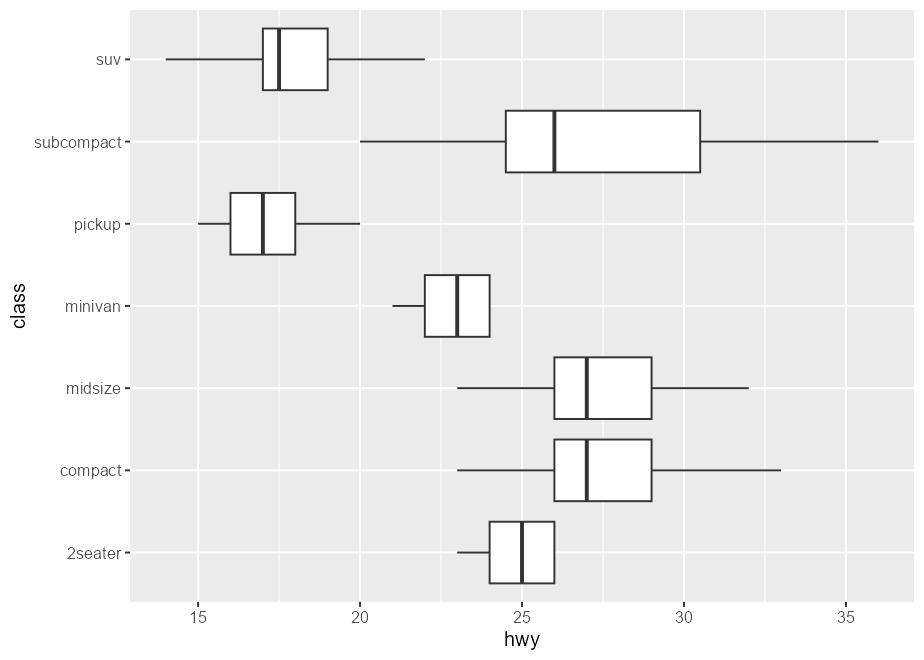Problem inspired by https://github.com/tidyverse/ggplot2/issues/4892. Note that this is also possible in “vanilla” ggplot. Following our earlier discussion of after_stat(): # Suppress warning from mapping to outliers aesthetic update_geom_defaults("boxplot", list(outliers = NULL)) ggplot(mpg, aes(hwy, class)) + geom_boxplot( # Equivalent effect of modifying the after-stat data aes(outliers = after_stat(list(NULL))) )### 3) Not just ggproto The method argument of ggtrace_*() workflow functions can be (almost) any function-like object called during the rendering of a ggplot. set.seed(2023) # Example from ?stat_summary summary_plot <- ggplot(mtcars, aes(mpg, factor(cyl))) + geom_point() + stat_summary(fun.data = "mean_cl_boot", colour = "red", linewidth = 2, size = 3) summary_plot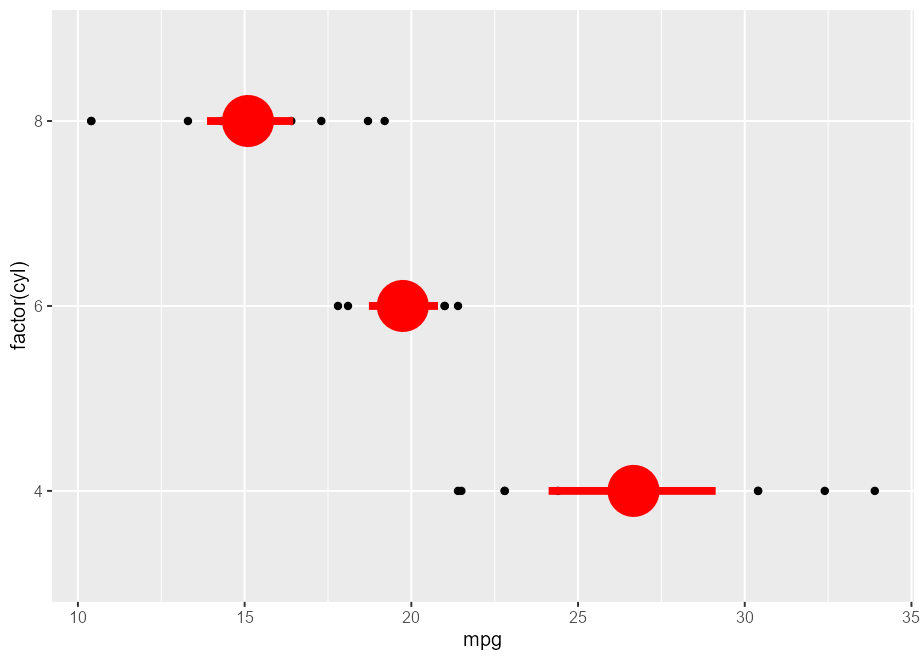ggtrace_inspect_args(x = summary_plot, method = mean_cl_boot) #>$x
#>   22.8 24.4 22.8 32.4 30.4 33.9 21.5 27.3 26.0 30.4 21.4
ggtrace_inspect_return(x = summary_plot, method = mean_cl_boot)
#>          y     ymin     ymax
#> 1 26.66364 24.11727 29.19159

ggtrace_highjack_return(
x = summary_plot, method = mean_cl_boot,
value = quote({
data.frame(y = 50, ymin = 25, ymax = 75)
})
)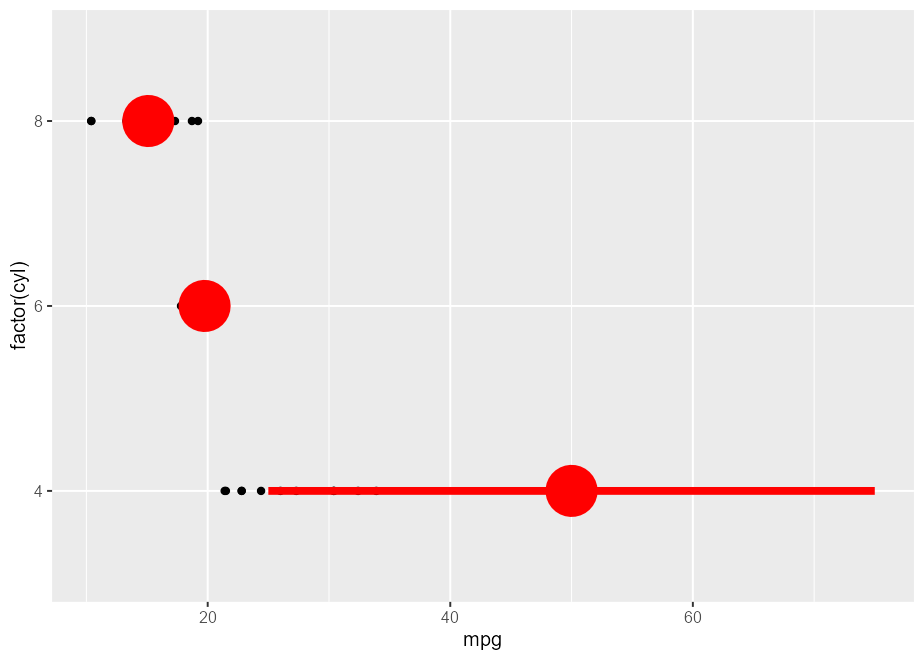### 4) Visually crop polar coordinate plots

Here’s a plot in polar coordinates:

polar_plot <- ggplot(mtcars, aes(hp, mpg)) +
geom_point() +
geom_smooth(method = "lm", formula = y ~ x) +
expand_limits(y = c(0, 60)) +
coord_polar(start = 0, theta = "y")

polar_plot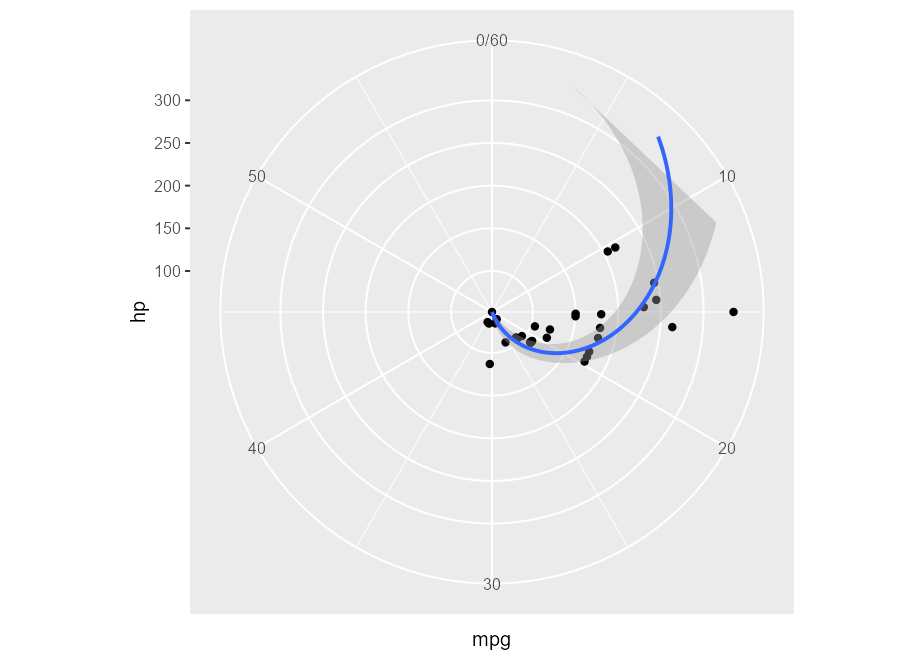We can clip the plot panel by highjacking the Layout$render() method using the generic workflow function with_ggtrace(): with_ggtrace( x = polar_plot + theme(aspect.ratio = 1/.48), method = Layout$render,
trace_steps = 5L,
trace_expr = quote({
panels[] <- editGrob(panels[], vp = viewport(xscale = c(.48, 1)))
}),
out = "g"
)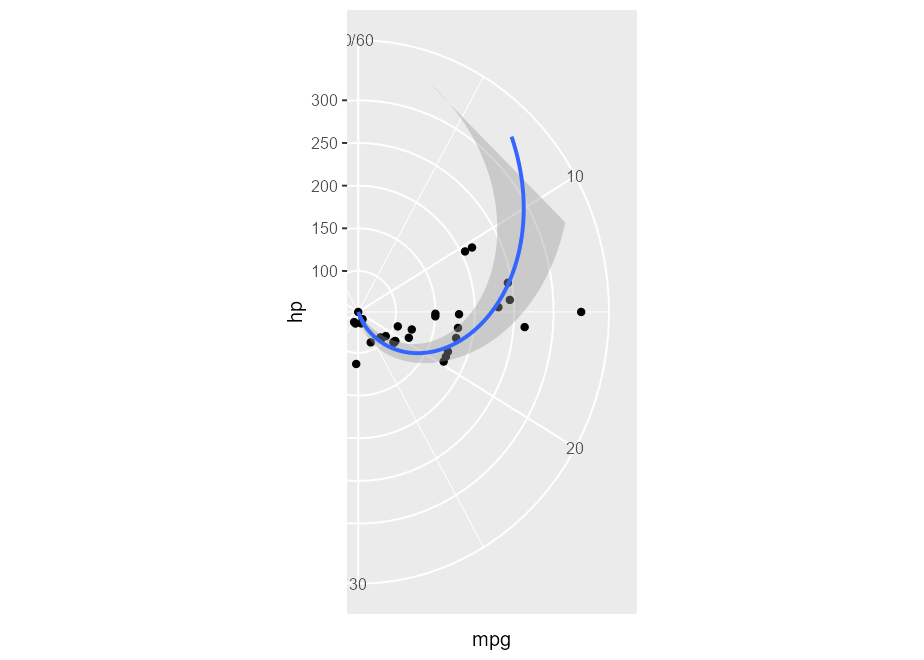Inspired by a twitter thread. See implementation in MSBMisc::crop_coord_polar().

### 5) Highjack the drawing context

Flashy example adopted from my UseR! talk:

library(palmerpenguins)
flashy_plot <- na.omit(palmerpenguins::penguins) |>
ggplot(aes(x = species, y = flipper_length_mm)) +
geom_boxplot(aes(fill = species), width = .7) +
facet_wrap(~ year)
flashy_plotggtrace_highjack_return(
flashy_plot, Geom\$draw_panel, cond = TRUE,
value = quote({
circ <- circleGrob(y = .25 * ._counter_)
grobTree( editGrob(circ, gp = gpar(fill = linearGradient())),
editGrob(returnValue(), vp = viewport(clip = circ)) )
}))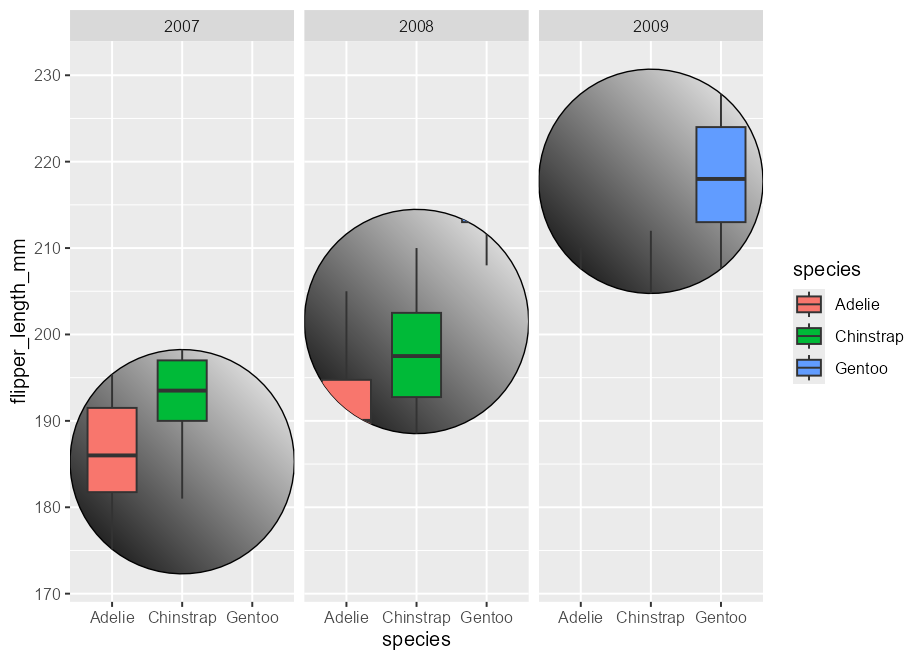Note the use of the special variable ._counter_, which increments every time a function/method has been called. See the reference section on tracing context for more details.